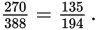# SAT Math Multiple Choice Question 405: Answer and Explanation

### Test Information

Question: 405

15. Plants are capable of cross-pollinating with related but different plants. This creates a hybrid plant. Sometimes, a hybrid plant is superior to the two different plants from which it was derived. This is known as "hybrid vigor." Scientists can examine the DNA of a plant to see if it is a hybrid. This can be valuable information because if the plant appears superior, it would be beneficial to develop more of these hybrids. An agricultural scientist examines an orchard that has several types of apple trees and orange trees to see which ones are hybrids. Some of the information she collected is shown in the table below.

 Apple Trees Orange Trees Total Hybrid 402 Non-hybrid 118 Total 628

The scientist wants to study the orange trees to check for hybrid vigor. If she chooses one orange tree at random, what is the probability that it will be a hybrid?

• A.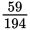• B.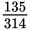• C.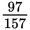• D.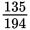Getting to the Answer: Because there are 402 hybrids in total, there are 402 - 132 = 270 orange trees that are hybrids, which means there are 270 + 118 = 388 orange trees in total. Now, find the probability that if the scientist selects one orange tree, it will be a hybrid. There are 388 orange trees total, and of those, 270 are hybrids, so the probability of picking a hybrid is